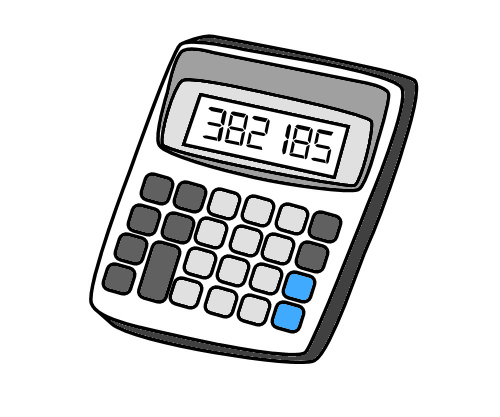## The KataRoy Osherove’s string calculator kata is a very simple kata designed (I believe) to demonstrate the basic TDD workflow.

The basic idea is to write a program (function?) that sums the integers in a string. “1,2,3” -> 6.

## Setting Up a Haskell Package for the Kata

I like to start by initialising an isolated package for each new project.

I used hsenv to create an isolated environment (similar to rbenv etc). Then added a cabal configuration file:

``````name:                stringcalc
version:             0.1.0.0
author:              Liam
build-type:          Simple
cabal-version:       >=1.8

library
exposed-modules:     StringCalc
-- other-modules:
hs-source-dirs:      src/
build-depends:       base ==4.6.*

test-suite tests
type:               exitcode-stdio-1.0
hs-source-dirs:     test/
main-is:            Spec.hs
build-depends:      base ==4.6.*
, hspec
, stringcalc
``````

This says that my project is library exporting one module, StringCalc, and that it has a test project in Spec.hs.

To get this to build / run I need to create the library src/StringCalc.hs:

``````module StringCalc where
``````

and the test file test/Spec.hs (these tests use the hspec test library):

``````import Test.Hspec
import Test.QuickCheck
import Control.Exception (evaluate)
import StringCalc

main :: IO ()
main = hspec \$ do

describe "Example Spec" \$ do
it "should pass" \$ do
(0 :: Int) `shouldBe` (0 :: Int)
``````

then build with cabal:

``````cabal configure --enable-tests
cabal install --enable-tests --only-dependencies
cabal build
cabal test
``````

## Implementing the Kata

### Step 1: “”

The first thing to do is to get the program to handle the empty string input.

``````main :: IO ()
main = hspec \$ do

describe "StringCalc" \$ do
it "should sum the empty string" \$ do
sumString "" `shouldBe` (0 :: Int)
``````

and then the implementation:

``````module StringCalc (sumString) where

sumString :: String -> Int
sumString "" = 0
``````

next deal with adding a single number:

``````describe "add single number" \$ do
it "should add positive number" \$ do
sumString "1" `shouldBe` (1 :: Int)

it "should add negative number" \$ do
sumString "-2" `shouldBe` (-2 :: Int)
``````
``````sumString :: String -> Int
sumString "" = 0
sumString s = sum \$ map rInt (split s)
where rInt :: String -> Int
``````

The next step is, “Allow the Add method to handle an unknown amount of numbers”:

``````describe "add two numbers" \$ do
describe "add 3 and 9" \$ do
it "should give 12" \$ do
sumString "3,9" `shouldBe` (12 :: Int)
``````
``````module StringCalc (sumString) where

sumString :: String -> Int
sumString "" = 0
sumString s = sum \$ map rInt (split s)
where rInt :: String -> Int

split :: String -> [String]
split "" = []
split (',':ss) = split ss
split s = [takeWhile isNotComma s :: String] ++ (split \$ dropWhile isNotComma s)
where isNotComma :: Char -> Bool
isNotComma c = c /= ','
``````

The sumString function now needs to be changed to use commas or newlines as the separator:

``````describe "with newline separators" \$ do
let input = "1\n2\n3"

it "should give 6" \$ do
sumString input `shouldBe` (6::Int)
``````
``````sumString :: String -> Int
sumString "" = 0
sumString s = sum \$ map rInt (split s)
where rInt :: String -> Int

split :: String -> [String]
split "" = []
split (',':ss) = split ss
split ('\n':ss) = split ss
split s = [takeWhile isNotSeparator s :: String] ++ (split \$ dropWhile isNotSeparator s)
where isNotSeparator :: Char -> Bool
isNotSeparator c = c /= ',' && c /= '\n'
``````

The final requirement is to support different delimeters. Here it is, with some refactoring:

``````module StringCalc (sumString) where

import Data.List
import Data.List.Split

hasExplicitDelimeter :: String -> Bool
hasExplicitDelimeter ('/':'/':s:'\n':ss) = True
hasExplicitDelimeter _                   = False

trimmed :: String -> String
trimmed input | hasExplicitDelimeter input = drop 4 input
| otherwise                  = input

sumString :: String -> Int
sumString "" = 0
sumString s = sum . map rInt \$ splitOn [guessDelimeter s] (trimmed s)

where rInt :: String -> Int